Function Repository Resource:

# PowerRule

See how to apply the power rule for derivatives to an expression

Contributed by: Wolfram|Alpha Math Team
 ResourceFunction["PowerRule"][f,x] applies the power rule for computing the derivative of f with respect to x, returned as an Inactive result.

## Details

ResourceFunction["PowerRule"] accepts the following options:
 "PrintRule" False print a statement of the power rule "PrintNapkin" False print the side "napkin" word needed for computing components of the power rule

## Examples

### Basic Examples (1)

Show the power rule for computing the derivative of 4x2 with respect to x:

 In:=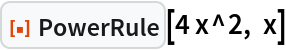Out=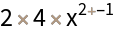### Scope (1)

The power rule can also be applied to expressions with negative exponents:

 In:=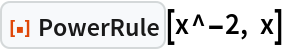Out=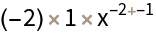### Options (2)

#### PrintNapkin (1)

Setting "PrintNapkin" to True prints a grid with the side "napkin" work needed to apply the power rule:

 In:=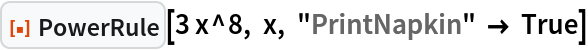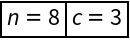Out=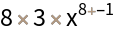#### PrintRule (1)

Setting "PrintRule" to True prints a general statement of the power rule for derivatives:

 In:=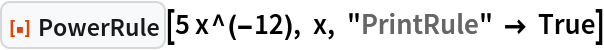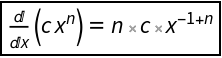Out=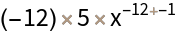## Publisher

Wolfram|Alpha Math Team

## Version History

• 1.0.0 – 17 April 2023

## Author Notes

To view the full source code for PowerRule, evaluate the following: